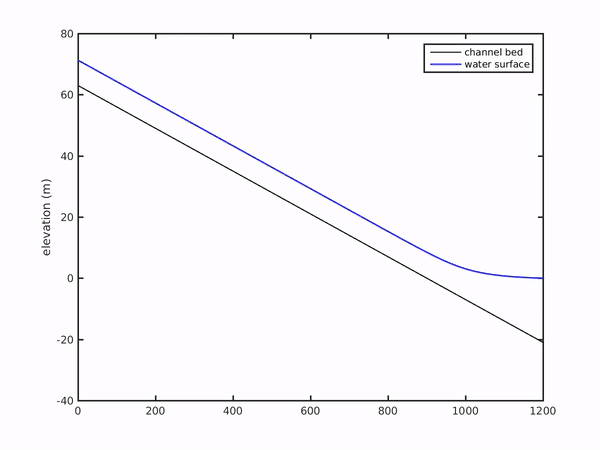# Building a simple delta numerical model: Part VI

This will be the final piece of the model that we need to get to have a working code for delta growth: the time routine. We will define a few more terms in order to set up the model to be able to loop through all the time steps and update the evolving delta.

T = 500; 						% yrs
timestep = 0.1; 				% timestep, fraction of years
t = T/timestep; 				% number of timesteps
dtsec = 31557600 * timestep;	% seconds in a timestep


T is the total time the model will be run for (in years), timestep is the fraction of year that will be simulated with each timestep, expressed in seconds as dtsec, and t is the number of timesteps to loop through. Now we simply take our block of code that we’ve built up to calculate all the propertyies of the delta (slope, sediment transport, deposition, etc.) and surround it with a for statement:

for i = 1:t
[S] = get_slope(eta, nx, dx); 							% bed slope at each node
[H] = get_backwater_fixed(eta, S, H0, Cf, qw, nx, dx); 	% flow depth
U = Qw ./ (H .* B0); 									% velocity
[qs] = get_transport(U, Cf, d50, Beta);
qsu = qs(1); 											% fixed equilibrium at upstream
[dqsdx] = get_dqsdx(qs, qsu, nx, dx, au);
[eta] = update_eta(eta, dqsdx, phi, If, dtsec);
end


To explain in words the above block, now, we are going to go through every timestep i and calculate the slope of the bed (eta) everywhere in the model domain, then we use this slope (and other defined parameters) to determine the flow depth and velocity through the entire delta. The velocity is used to calculate sediment transport, and the change in sediment transport over space produces a change in the channel bed elevation over time (i.e., the Exner equation). Finally, we return to the top of the loop to calculate a new slope based on our new bed.

That’s it! Our delta model is now complete and we can outfit it with all sorts of bells and whistles to test hypotheses about delta evolution in the natural (or experimental) world. A powerful tool indeed!

Below is a simple movie output from this model that shows the results of our hard work! The complete code for the delta model can be found here.Note that there is a small instability that grows at the front of the sediment wedge, this isn’t a huge problem depending on what you want to do with your model, but you can tweak dx and dt to make things run “smoother” (see the CFL number for more information).

This material is based upon work supported by the National Science Foundation (NSF) Graduate Research Fellowship under Grant No.145068 and NSF EAR-1427177. Any opinion, findings, and conclusions or recommendations expressed in this material are those of the author(s) and do not necessarily reflect the views of the National Science Foundation.

Updated: